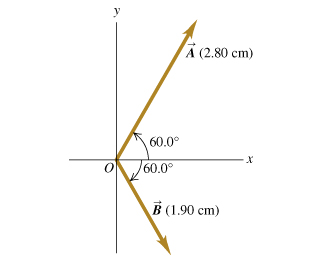# Problem: Vector A is 2.80 cm long and is 60.0B above the x-axis in the first quadrant. Vector cm is 1.90 cm long and is 60.0B below the x-axis in the fourth quadrant (the figure ). You may want to review (Pages 14 - 18) . For related problemsolving tips and strategies, you may want to view a Video Tutor Solution of Using components to add vectors.Use components to find the magnitude of A+B.Use components to find the magnitude of A - B.Use components to find the magnitude of B - A. Use components to find the direction of A+B.Use components to find the direction of A - B.Use components to find the direction of B - A.Sketch the vector addition C = A + B.Sketch the vector subtraction C = A - B.Sketch the vector subtraction C = B - A.

🤓 Based on our data, we think this question is relevant for Professor Lin's class at UNT.

###### Problem Details
Vector A is 2.80 cm long and is 60.0B above the x-axis in the first quadrant. Vector cm is 1.90 cm long and is 60.0B below the x-axis in the fourth quadrant (the figure).

You may want to review .

For related problemsolving tips and strategies, you may want to view a Video Tutor Solution of Using components to add vectors.

Use components to find the magnitude of A+B.

Use components to find the magnitude of A - B.

Use components to find the magnitude of B - A.

Use components to find the direction of A+B.

Use components to find the direction of A - B.

Use components to find the direction of B - A.

Sketch the vector addition C = A + B.

Sketch the vector subtraction C = A - B.

Sketch the vector subtraction C = B - A.